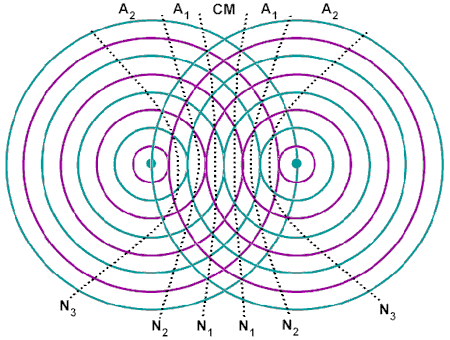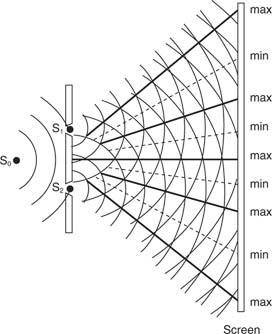# Circular interference from the equation of a hyperbola

• dimestorelaser
In summary, the hyperbolae shown in the diagram are the loci of maximum constructive interference, and hyperbolic radio navigation systems use these patterns to calculate position.

#### dimestorelaser

Hi all,

I had a question that I can't seem to find an answer too.
I was hoping people could point me in the right direction, or let me know if there is an "easy" method.

It has to do with the classic example of two stones in water producing constructive and destructive interference patterns, which create a hyperbola.

If we are given the closest hyperbola created by the interference patterns. Is there an "easy" way...to generate the equations for the other hyperbola that are also created?

Generally I have just been plotting some circles and "guessing" at the other ones. However it seems like there should be a pretty easy method...since they all have the same focus right?

For example, I am trying to create the other hyperbolic arcs created at the same time is this mildly complex hyperbola:

$y^2 = 4x^2 + 5x +632378$

Here is the wolframalpha of the equation:
http://www.wolframalpha.com/input/?i=y^2+=+4*x^2++5*x++632378
I would appreciate any feedback. Thank you!

There are formulas to get the focal points.

The manual way to find them: substitute ##z=x+\frac{5}{4} ## or equivalently ## x=z-\frac{5}{4}##, then ##4x^2 + 5x = 4z^2 -\frac{25}{16}##.
That allows to convert it to standard form easily and then you get your focal points. It is possible to pack everything together in formulas, too, even for rotated hyperbolas (where you get xy terms).

Hi thanks for the response,

Im not sure I was clear in what I was asking.

I know how to get the focus of the equation, that's not the question. My question is about the set of hyperbolas created by those foci. Consider a two point source at the locations of the foci, how do I determine the equations of ALL the hyperbolas associated with those foci. (Assuming that we have wavelength of 1).In the above picture...If I know the equation of N3...can I easily determine the equations for N2 and N1?

I appreciate the response. Thank you.

dimestorelaser said:
how do I determine the equations of ALL the hyperbolas associated with those foci. (Assuming that we have wavelength of 1).
The differences in distance to those foci have to be ... a-2, a-1, a, a+1, a+2, ... where a is some real number between 0 and 1, describing the relative phases of the emitters.

In the above picture...If I know the equation of N3...can I easily determine the equations for N2 and N1?
Calculate the distance difference for N3 and the focal points, reduce the distance difference by 1, 2, ... wavelengths.

HI thanks for the help, I appreciate it.

Would you mind working out the example I gave? I would help me to understand what you are saying. Thank you,

Hi, I think there is an error in your drawing - the loci of "maximum constructive interference" should be straight lines.
This diagram is from "Young's Experiment" on double slit interference.bubsir said:
Hi, I think there is an error in your drawing - the loci of "maximum constructive interference" should be straight lines.
This diagram is from "Young's Experiment" on double slit interference.

The loci are hyperbolae, hence the Hyperbolic Radio Navigation Systems. The Young's slits pattern that you show is an approximation, and only holds when the distances are greater than the separation. Note that the pattern in the original diagram also gives straight lines, well outside the region of the two sources.

Nevermind...I realized its actually quite simple.

## 1. What is circular interference from the equation of a hyperbola?

Circular interference from the equation of a hyperbola refers to the interference pattern that occurs when a circular wave is reflected off a hyperbolic surface. This interference pattern is characterized by concentric circles with varying intensities of light or other waves.

## 2. How is circular interference from the equation of a hyperbola different from other types of interference?

Circular interference from the equation of a hyperbola is unique because it is caused by the specific shape of the hyperbolic surface. Other types of interference, such as diffraction or refraction, occur due to different properties of the surface or medium.

## 3. What factors affect the circular interference pattern from a hyperbola?

The circular interference pattern from a hyperbola is affected by the wavelength of the incident wave, the angle of incidence, and the curvature of the hyperbolic surface. These factors can determine the size and intensity of the concentric circles in the interference pattern.

## 4. Can circular interference from the equation of a hyperbola be observed in real-life situations?

Yes, circular interference from the equation of a hyperbola can be observed in various natural and man-made situations. Examples include the reflection of sunlight off the surface of a lake or the interference patterns created by sound waves bouncing off a curved wall.

## 5. What practical applications does circular interference from the equation of a hyperbola have?

Circular interference from the equation of a hyperbola has various practical applications, such as in optics, acoustics, and radar technology. It can also be used in the design of reflective surfaces, such as satellite antennas, to control and manipulate interference patterns for specific purposes.